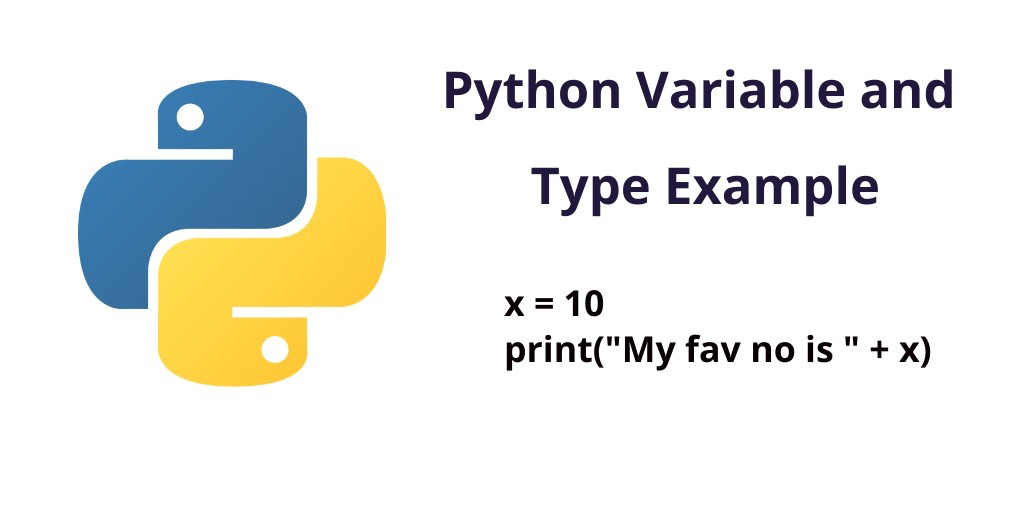Python 01-Jun-2022

### Python Variable and Type Example

In this python variable and it’s type example tutorial. You will learn what is variable in python, what types of variable in python and how to create and use variables in python.

## Python Variable and Type with example

Here you will learn how to create/declare variables in python and also you will learn how many types of declaring the variable in python.

### What is the variable in python?

Basically a variable in python is nothing, but it only holds the data or any values in it. There is no special command to make it. You can easily change it value on runtime.

Before we talk more about python variable and it’s types, we should know the important things or rules for creating variables in the python programming language.

#### Rules for creating a variable in python

Rule 1: A variable name must begin with a letter or the underscore character
Rule 2: A variable name cannot begin with a number
Rule 3: The python variable name can only contain alpha-numeric characters and underscores (A-z, 0-9, and _ )
Rule 4: A Variable names are case-sensitive Like name, Name and NAME are three different variables

### Python declare/create variable

Here first we will create/declare a variable. And also assign value to it.

For example:

```x = 10
print("My fav no is " + x)```

### Change variable value in python

We will create a variable and change the value of this variable at runtime.

```x = 10
print("My fav no is " + x)
x = 15
print("My fav no is " + x) ```

### Assigning a single value to multiple variables

Also Python allows to assign a single value to several variables simultaneously.

For example:

``` x = y = z = 10
print(x)
print(y)
print(z)        ```

### Assigning a different values to multiple variables

``` x, y, z = 5, "string", 99
print(a)
print(b)
print(c)         ```

When you create/declare a variable in Python. So you do not need to declare its type. Python itself detects what type of value store in it. Means variable has numbers or is a string or float value is value, etc.

Note:- And if you do not assign any value type value to it, then it will throw the exception.

For example:

``` #int value
myint = 7
print(myint)

#float value
myfloat = 7.0
print(myfloat)

#string vlaue
mystring = "hello"
print(mystring)

#invalid assigment
invalid = 5 + "5"
print(invalid)```

### How many types of variable in python?

Variables in Python programming are of two types, such as Local & Global Variables.

#### Local Variable

Which variables are made inside the function. They are called local variables. Local variables can be used only inside the function.

For example:

```def valfun():
x = "great"
print("Python is " + x)
valfun()```

#### Global Variable

Which variables are made outside the function. They are called global variables. Global variables can be used both inside and outside the function.

For example:

```x = "great"
def valfun():
print("Python is " + x)
valfun()```

### Global Keyword

Generally, when you create a variable inside any function, that is local variable. And you can not use this variable outside of the function.

You can create/define a global variable inside the function by using the global keyword.

For example:

```def valfun():
global x
x = "powerful"
valfun()
print("Python is " + x)```